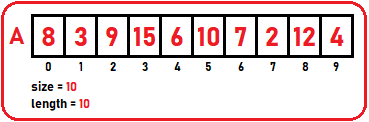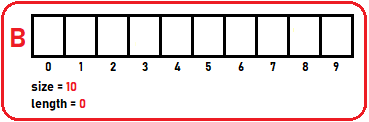# Array Reverse and Shift Operations in C

## Array Reverse and Shift Operations in C Language:

In this article, I am going to discuss How to Perform Array Reverse and Shift Operations in C Language with Examples. Please read our previous article, where we discussed Array Basic Operations with Examples.

##### Array Reverse Operation:

For reversing an array, we have two methods:

##### 1st Method:

In the first method, we have an array of filled elements:We will create an auxiliary array ‘B’ (extra array) so that we can store all the elements of array ‘A’:We will copy all the elements from the actual array A to auxiliary array B in reverse order. So, from the last element will copy.In the above image, we can clearly see the order in which we copied elements from array ‘A’ to ‘B’. Now we will copy these elements from array ‘B’ back to array ‘A’. Corresponding elements or same position elements will be copied. In this method, we require an extra array.

##### Let’s implement this approach in our code.

We have two arrays: original and auxiliary array. To copy the elements from array ‘A’ to array ‘B’, we require some variables which one variable point on the last element of array ‘A’ and keep decrementing till first element and another variable point on the first element of array ‘B’ and keep incrementing till it reaches the last element, In the for loop, we will add below statement:

B[j] = A[i];

Let’s see step by step:

Step1: we initialized i = last index of A and j = 0th index of B.Here 4 is copied to B .

Step2: Now, decrement i to 8th index of A and increment j to 1st index of B.Here 12 is copied to B .

Step3: In the same manner, the whole array will be copied in reverse order:Step4: Now, we have to copy all elements from array ‘B’ to array ‘A’ in the same order.

Step5: For that, we have to point i to A  and j to B :Step6: Iterate through all the elements with the help of for loop and copy one by one element from array ‘B’ to array ‘A’:This method requires n + n time:

Time Complexity: O(n)

##### Array Reverse() Operation Code in C Language:
```void Reverse(struct Array *arr){
int *B;
int i,j;

B=(int *)malloc(arr->length*sizeof(int));
for(i=arr->length-1,j=0;i>=0;i--,j++)
B[j]=arr->A[i];
for(i=0;i<arr->length;i++)
arr->A[i]=B[i];
}

```
##### 2nd Method:

In the second method, we can scan from two ends of an array and interchange the elements or swap the elements. For example, we have an array of size 10:So, 0th element swap with 9th element, i.e. i = 0 and j = 9:1st element swap with 8th element, i.e. i = 1 and j = 8:2nd element swap with 7th element, i.e. i = 2 and j = 7:And so on…##### Reverse2() Code:
```void swap(int *x,int *y){
int temp=*x;
*x=*y;
*y=temp;
}

void Reverse2(struct Array *arr){
int i,j;
for(i=0,j=arr->length-1;i<j;i++,j--){
swap(&arr->A[i],&arr->A[j]);
}
}
```
##### Array Left / Right Shift:

Let look at the left shift operation. Let’s take a small size array of size 5:So, what does it mean by left shift? We want to shift all the elements on the left-hand side.Above is the demonstration of left shifting. We have lost one element in the above left shifting.

1. In left shifting, we will lose the first element.
2. In right shifting, we will lose the last element.
3. In right shifting, we will shift all the elements towards the right-hand side of the array. It is the same as left shifting.

Time Complexity: O (n)

##### Array Left / Right Rotate:

In the left or right rotation, the element will not be deleted from the array, if it is left rotating, then the first element will move to the last position, or if it is right rotating, then the last element will move to the first position. Below is the array of size 5:Below is the example of left rotation in the above array:Shifting means removing either the first or last element from the array. Rotation means shifting the first element to the last position or shifting the last element to the first position in the array.

Time Complexity: O (n)

##### Use of Array Rotation and Shifting:

On display boards, we find that the advertisements are scrolled on the display board.So, this can be displayed like this. Now we want to show it to us as a moving or scrolling display. So, what do we have to do to move the above display? Just remove the first element on and shift all the elements towards left and when all letters have shifted towards left, add those letters from last and continue this step again and again… So, this is the logic used for the LED display board.

##### Full Code in C Language:
```#include<stdio.h>
#include<stdlib.h>
struct List{
int B;
int size;
int length;
};

void Display(struct List list){
int i;
printf("\nElements are\n");
for(i=0;i<list.length;i++)
printf("%d ",list.B[i]);
}

void swap(int *x,int *y){
int temp=*x;
*x=*y;
*y=temp;
}

void Reverse(struct List *list){
int *C;
int i,j;

C=(int *)malloc(list->length*sizeof(int));
for(i=list->length-1,j=0;i>=0;i--,j++)
C[j]=list->B[i];
for(i=0;i<list->length;i++)
list->B[i]=C[i];
}

void Reverse2(struct List *list){
int i,j;
for(i=0,j=list->length-1;i<j;i++,j--){
swap(&list->B[i],&list->B[j]);
}
}

int main()
{
struct List list1={{2,3,9,16,18,21,28,32,35},10,9};

printf("Before Reverse:");
Display(list1);

printf("\n\n");

Reverse(&list1);

printf("After Reverse:");
Display(list1);

return 0;
}
```
###### Output:In the next article, I am going to discuss Checking if Array is Sorted in C Language with Examples. Here, in this article, I try to explain how we can perform Array Reverse and Shift Operations in C Language with Examples and I hope you enjoy this Array Reverse and Shift Operations in C Language with Examples article.